# Abstract

A Hyperbolic Shear Deformation Theory (HPSDT) taking into account transverse shear deformation effects, is used for the static flexure analysis of thick isotropic beams. The displacement field of the theory contains two variables. The hyperbolic sine function is used in the displacement field in terms of thickness coordinate to represent shear deformation. The transverse shear stress can be obtained directly from the use of constitutive relations, satisfying the shear stress-free boundary conditions at top and bottom of the beam. Hence, the theory obviates the need of shear correction factor. Governing differential equations and boundary conditions of the theory are obtained using the principle of virtual work. General solutions of thick isotropic simply supported, cantilever and fixed beams subjected to uniformly distributed and concentrated loads are obtained. Expressions for transverse displacement of beams are obtained and contribution due to shear deformation to the maximum transverse displacement is investigated. The results of the present theory are compared with those of other refined shear deformation theories of beam to verify the accuracy of the theory.

hyperbolic shear deformation theory; static flexure; general solution of beams; shear contribution factor

A refined shear deformation theory for flexure of thick beams

Yuwaraj M. Ghugal* * Author email: ghugal@rediffmail.com ; Rajneesh Sharma

Department of Applied Mechanics, Government College of Engineering, Aurangabad-431005, Maharashtra State  India

ABSTRACT

A Hyperbolic Shear Deformation Theory (HPSDT) taking into account transverse shear deformation effects, is used for the static flexure analysis of thick isotropic beams. The displacement field of the theory contains two variables. The hyperbolic sine function is used in the displacement field in terms of thickness coordinate to represent shear deformation. The transverse shear stress can be obtained directly from the use of constitutive relations, satisfying the shear stress-free boundary conditions at top and bottom of the beam. Hence, the theory obviates the need of shear correction factor. Governing differential equations and boundary conditions of the theory are obtained using the principle of virtual work. General solutions of thick isotropic simply supported, cantilever and fixed beams subjected to uniformly distributed and concentrated loads are obtained. Expressions for transverse displacement of beams are obtained and contribution due to shear deformation to the maximum transverse displacement is investigated. The results of the present theory are compared with those of other refined shear deformation theories of beam to verify the accuracy of the theory.

Keywords: hyperbolic shear deformation theory, static flexure, general solution of beams, shear contribution factor.

1 INTRODUCTION

The Bernoulli-Euler elementary theory of bending (ETB) of beam disregards the effect of the shear deformation. The theory is suitable for slender beams and is not suitable for thick or deep beams since it is based on the assumption that the transverse normal to the neutral axis remains so during bending and after bending, implying that the transverse shear strain is zero. Since the theory neglects the transverse shear deformation, it underestimates deflections and overestimates the natural frequencies in case of thick beams, where shear deformation effects are significant.

The first order shear deformation theory (FSDT) of Timoshenko  includes refined effects such as the rotatory inertia and shear deformation in the beam theory. Timoshenko showed that the effect of transverse shear is much greater than that of rotatory inertia on the response of transverse vibration of prismatic bars. In this theory transverse shear strain distribution is assumed to be constant through the beam thickness and thus requires shear correction factor to appropriately represent the strain energy of deformation. Cowper  has given refined expression for the shear correction factor for different cross-sections of the beam.

NOMENCLATURE

A Cross sectional area of beam; b Width of beam in y direction; E, G, µ Elastic constants of the material; E Young's modulus; G Shear modulus; h Thickness of beam; I Moment of inertia of cross section of beam; L Span of the beam; q Intensity of uniformly distributed transverse load; u Axial displacement in x direction; w Transverse displacement in z direction; x, y, z Rectangular Cartesian coordinates; µ Poisson's ratio of the beam material; σ x Axial stress in x direction;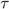xz Transverse shear stress in zx plane; Φ Unknown function associated with the shear slope.

ABBREVIATIONS

ETB Elementary Theory of Beam-bending FSDT First-order Shear Deformation Theory HSDT Higher-order Shear Deformation Theory TSDT Trigonometric Shear Deformation Theory PSDT Parabolic Shear Deformation Theory HPSDT Hyperbolic Shear Deformation Theory UDL Uniformly distributed load

The discrepancies in the elementary theory of beam bending and first order shear deformation theory forced the development of higher order or equivalent refined shear deformation theories. Levinson , Bickford , Rehfield and Murthy , Krishna Murty , Baluch, et.al , Bhimaraddi and Chandrashekhara  presented parabolic shear deformation theories assuming a higher variation of axial displacement in terms of thickness coordinate. These theories satisfy shear stress free boundary conditions on the top and bottom surfaces of the beam and thus obviate the need of shear correction factor. Kant and Gupta , and Heyliger and Reddy  presented higher order shear deformation theories for the static and free vibration analyses of shear deformable uniform rectangular beams.

The theories based on trigonometric and hyperbolic functions to represent the shear deformation effects through the thickness is the another class of refined theories. Vlasov and Leont'ev  and Stein  developed refined shear deformation theories for thick beams including sinusoidal function in terms of thickness coordinate in the displacement field. However, with these theories shear stress free boundary conditions are not satisfied at top and bottom surfaces of the beam. This discrepancy is removed by Ghugal and Shimpi  and developed a variationally consistent refined trigonometric shear deformation theory for flexure and free vibration of thick isotropic beams. Ghugal and Nakhate  obtained the general bending solutions for thick beams using variationally consistent refined trigonometric shear deformation theory. Ghugal and Sharma  developed the variationally consistent hyperbolic shear deformation theory for flexural analysis of thick beams and obtained the displacements, stresses and fundamental frequencies of flexural mode and thickness shear modes from free vibration of simply supported beams.

In this paper, a variationally consistent hyperbolic shear deformation theory previously developed by Ghugal and Sharma  for thick beams is used to obtain the general bending solutions for thick isotropic beams. The theory is applied to uniform isotropic solid beams of rectangular cross-section for static flexure with various boundary and loading conditions. The results are compared with those of elementary, refined and exact beam theories available in the literature to verify the credibility of the present shear deformation theory.

2 THEORETICAL FORMULATION

The variationally correct forms of differential equations and boundary conditions, based on the assumed displacement field are obtained using the principle of virtual work. The beam under consideration occupies the following region: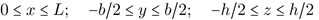where x, y, z are Cartesian coordinates, L is the length, b is the width and h is the total depth of beam. The beam is subjected to transverse load of intensity q(x) per unit length of the beam. The beam can have any meaningful boundary conditions.

2.1 The displacement field

The displacement field of the present beam theory is of the form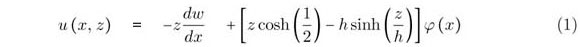Here u and w are the axial and transverse displacements of the beam center line in the x and z directions respectively. The first term in Eqn (1) is the axial displacement according to the elementary theory of beam bending (ETB) due to Bernoulli-Euler which is linear through the thickness of the beam the second term in the bracket is the displacement due to transverse shear deformation, which is assumed to be hyperbolic sine function in terms of thickness coordinate, which is non-linear in nature through the thickness of beam. The hyperbolic sine function is assigned according to the shearing stress distribution through the thickness of the beam. The φ(x) is an unknown function to be determined and is associated with the rotation of the cross-section of the beam at neutral axis.Stresses

One dimensional constitutive laws are used to obtain normal bending and transverse shear stresses. These stresses are given bywhere E and G are the elastic constants of beam material.

2.2 Governing equations and boundary conditions

Using the expressions (3) through (5) for strains and stresses and dynamic version of principle of virtual work, variationally consistent governing differential equations and boundary conditions for the beam under consideration are obtained. The principle of virtual work when applied to the beam leads towhere the symbol δ denotes the variational operator. Employing Green's theorem in Eqn. (6) successively, we obtain the coupled Euler-Lagrange equations which are the governing differential equations of the beam and the associated boundary conditions of the beam. The governing differential equations obtained are as follows:where A0, B0 and C0 are the constants as given in Appendix and the associated boundary conditions obtained are as follows:Thus the variationally consistent governing differential equations and the associated boundary conditions are obtained. The static flexural behavior of beam is given by the solution of these equations and simultaneous satisfaction of the associated boundary conditions.

2.3 The general solution for the static flexure of beam

Using the governing equations (7) and (8) for static flexure of beam, the general solution for w(x) and Φ(x) can be obtained. By integrating and rearranging the first governing equation (Eqn. 7) one can get following equationwhere Q(x) is the generalized shear force for the beam under consideration and it is given by Q(x) = q dx + C1. The second governing equation (Eqn. 8) can be written as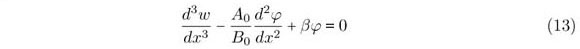Using Eqn. (12) and Eqn. (13), a single differential equation in terms of Φ can be obtained as follows.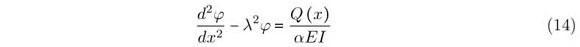where the constant α, β and λ used in Eqn. (13) and Eqn. (14) are given in Appendix. The general solution of above Eqn. (14) is given by:The general solution for transverse displacement (w) can be obtained by substituting the expression for φ(x) in Eqn. (13) and integrating thrice with respect to x. The solution iswhere C1 - C6 are the arbitrary constants of integration and can be obtained by imposing natural (forced) and kinematic (geometric) boundary conditions of beams.

3 ILLUSTRATIVE EXAMPLES

3.1 Example 1: Simply supported beam with uniformly distributed load q

A simply supported beam with rectangular cross section (b × h) is subjected to uniformly distributed load q over the span L at surface z =  h/2 acting in the downward z direction. The origin of beam is taken at left end support i.e. at x = 0. The boundary conditions associated with simply supported beam are as follows.The boundary condition, Φ = 0 at x = L/2 is used from the condition of symmetry of deformation, in which the middle cross section of the beam must remain plane without warping (see Timoshenko ). Applying appropriate boundary conditions from (19) and (20) in general solutions of the beam the final expressions for Φ(x) and w(x) are obtained as follows:The maximum transverse displacement at x = L/2 obtained from Eqn. (20) is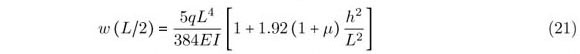3.2 Example 2: Simply supported beam with central concentrated load P

A simply supported beam with rectangular cross section (b × h) subjected to concentrated load P at mid span i.e. at x = L/2 at surface z = h/2. The origin beam is taken at left end support i.e. at x = 0. The boundary conditions associated with simply supported beam with a concentrated load are given as:From the condition of symmetry, the middle cross-section of the beam must remain normal and plane, hence shear rotation, Φ = 0 at x = L/2 . Using these boundary conditions, in the region (0 = x = L/2) of beam, the general expressions for Φ(x) and w(x) are obtained as follow: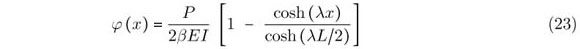The maximum transverse displacement at x = L/2 obtained from Eqn. (24) is3.3 Example 3: Cantilever beam with uniformly distributed load q

A cantilever beam with rectangular cross section (b × h) is subjected to uniformly distributed load q at surface z = h/2 acting in the z direction. The origin of beam is taken at free end i.e. at x = 0 and it is fixed or clamped at x = L. The boundary conditions associated with cantilever beam are as given:Using these boundary conditions the general expressions for Φ(x) and w(x) are obtained from the general solution as follows:The maximum transverse displacement at free end (x = 0) obtained from Eqn. (28) is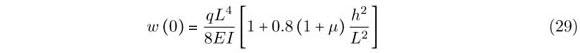3.4 Example 4: Cantilever beam with concentrated load P at free end

A cantilever beam with rectangular cross section (b × h) is subjected to concentrated load P at free end i.e. at x = L at surface z = h/2 acting in the z direction. The origin of the beam is taken at fixed end i.e. at x = 0. The boundary conditions associated with cantilever beam are as given: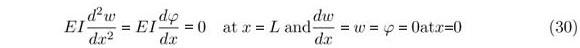Using these boundary conditions in general solution of the beam, the general expressions for Φ(x) and w(x) are obtained as follows:The maximum transverse displacement at free end (x = L) obtained from Eqn. (32) is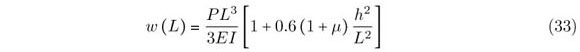3.5 Example 5: Fixed-fixed (Clamped-clamped) beam with uniformly distributed load q

A fixed-fixed beam with rectangular cross section (b × h) is subjected to uniformly distributed load q at surface z = h/2. The origin of the beam is taken at left end fixed/clamped support i.e. x = 0. The boundary conditions associated with this beam are as follows:Using the appropriate boundary conditions, from the set given by Eqn. (34), in general solution of the beam, the general expressions for Φ(x) and w(x) are obtained as follows:The maximum transverse displacement at center of the beam (x = L/2) obtained from Eqn. (36) is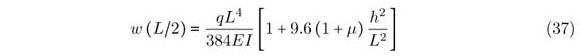3.6 Example 6: Fixed-fixed beam with central concentrated load P

A fixed-fixed beam with rectangular cross section (b × h) is subjected to concentrated load P at mid span at surface z = h/2. The origin of the beam is taken at left end fixed/clamped support i.e. x = 0. The boundary conditions associated with this beam are as follows: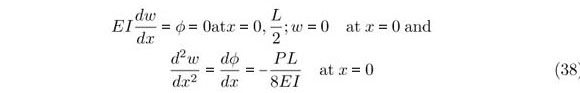Using these boundary conditions, in the region (0 = x = L/2) of beam, the general expressions for Φ(x) and w(x) are obtained as follow: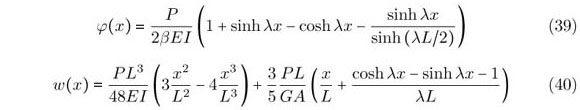The maximum transverse displacement at center of the beam (x = L/2) obtained from Eqn. (40) is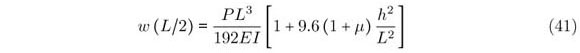While obtaining the expressions for maximum transverse displacement (deflection) in the above examples it is observed that the quantity λL is very large and therefore tanh λL ~ 1, 1/λL ~ 1/(λL)2~ 0 and sinh λL ~ cosh λL. For problems of practical interest this is a very good approximation.

4 RESULTS

In expressions of maximum transverse displacement the first term in the bracket is the displacement contribution according to the classical Bernoulli-Euler beam theory and the second term represents the effect of transverse shear deformation. These expressions can be written in the generalized form as follows: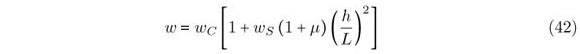In the above equation wc is the transverse displacement according to the classical Bernoulli-Euler beam theory and ws is the proportionality constant due to transverse shear deformation effect. In Tables 1 through 3 the values of ws for different beam problems are compared with those of other refined theories.

The numerical results shown in Table 1 for simply supported beam with uniform load and concentrated load indicate that the results of proportionality constant (ws) due to shear deformation effect according to present theory (HPSDT) are identical to those of beam theories of Timoshenko (FSDT), Levinson (HSDT), Bhimaraddi and Chandrashekhara (PSDT), and Ghugal and Nakhate (TSDT). The results of these theories are closed to exact value in case of simply supported beam with uniform load. In case of cantilever and fixed-fixed beams with uniform load Levinson's variationally inconsistent beam theory yields higher values of ws than those obtained by present theory and other refined theories as shown in Tables 2 and 3. However, for cantilever beam with concentrated load Levinson's theory gives the exact value of this constant (see Table 2). In case of beam with both the ends fixed, it is observed that the constant of proportionality due to shear deformation is independent of loading conditions.

5 CONCLUSIONS

In this paper a hyperbolic shear deformation theory has been used to obtain the bending solutions for thick homogeneous, isotropic, statically determinate and indeterminate beams. General solutions for the transverse displacement and rotation are presented for transversely loaded beams with various end conditions. Expressions for maximum transverse displacements are deduced from the general solutions of the thick beams. The effect of transverse shear deformation on the bending solutions of thick beams can be readily observed from the analytical expressions presented for the transverse displacement. The values of transverse displacement contribution (ws) due to transverse shear deformation effect obtained by present theory are found to be identical to those of first order shear deformation theory of Timoshenko with the shear correction factor equal to 5/6. The present theory requires no shear correction factor. The accuracy of present theory is verified by comparing the results of other refined theories and the exact theory.

In revised form 21 Dec 2010

APPENDIX

The constants A0, B0 and C0 appeared in governing equations (7) and (8) and boundary conditions given by equations (9) - (11) are as follows:The constants α, β and λ appeared in Eqns. (13) and (14) are as follows:•  M. H. Baluch, A. K. Azad, and M. A. Khidir. Technical theory of beams with normal strain. J. Engg. Mech., ASCE, 110(8):12331237, 1984.
•  A. Bhimaraddi and K. Chandrashekhara. Observations on higher order beam theory. J. Aerospace Engineering, ASCE, 6(4):408413, 1993.
•  W. B. Bickford. A consistent higher order beam theory. Dev. in Theoretical and Applied Mechanics, SECTAM, 11:137150, 1982.
•  G. R. Cowper. The shear coefficients in Timoshenko beam theory. J. Applied Mechanics, ASME, 33(2):335340, 1966.
•  Y. M. Ghugal and V. V. Nakhate. Flexure of thick beams using trigonometric shear deformation theory. The Bridge and Structural Engineer, 39(1):117, 2009.
•  Y. M. Ghugal and R. Sharma. A hyperbolic shear deformation theory for flexure and free vibration of thick beams. International Journal of Computational Methods, 6(4):585604, 2009.
•  Y. M. Ghugal and R. P. Shimpi. A trigonometric shear deformation theory for flexure and free vibration of isotropic thick beams. In Structural Engineering Convention, SEC-2000, pages 255263, Bombay, India, 2000. IIT.
•  P. R. Heyliger and J. N. Reddy. A higher order beam finite element for bending and vibration problems. J. Sound and Vibration, 126(2):309326, 1988.
•  T. Kant and A. Gupta. A finite element model for a higher order shear deformable beam theory. J. Sound and Vibration, 125(2):193202, 1988.
•  A. V. Krishna Murty. Towards a consistent beam theory. AIAA Journal, 22(6):811816, 1984.
•  M. Levinson. A new rectangular beam theory. J. Sound and Vibration, 74(1):8187, 1981.
•  L. W. Rehfield and P. L. N. Murthy. Toward a new new engineering theory of bending: fundamentals. AIAA J., 20(5):693699, 1982.
•  M. Stein. Vibration of beams and plate strips with three dimensional flexibility. J. App. Mech., ASME, 56(1):228231, 1989.
•  S. P. Timoshenko. On the correction for shear of the differential equation for transverse vibrations of prismatic bars. Philosophical Magazine Series, 6:742746, 1921.
•  S. P. Timoshenko. Strength of Materials, Part 1 Elementary Theory and Problem CBS Publishers and distributors, New Delhi, India, 3rd edition, 1986. Chapter 5.
•  S. P. Timoshenko and J. N. Goodier. Theory of Elasticity McGraw-Hill, Singapore, 3rd edition, 1970.
•  B. Venkatraman and S. A. Patel. Structural Mechanics with Introduction to Elasticity and Plasticity McGraw-Hill Book Company, New York, 1970. Chapter 11.
•  V. Z. Vlasov and U. N. Leont'ev. Beams, Plates, and Shells on Elastic Foundations Moskva, 1966. Translated from the Russian by Barouch, A., and Pelz, T., Israel Program for Scientific Translation Ltd., Jerusalem, Chapter 1.
• *
Author email:

# Publication Dates

• Publication in this collection
15 June 2011
• Date of issue
June 2011

• Reviewed
21 Dec 2010